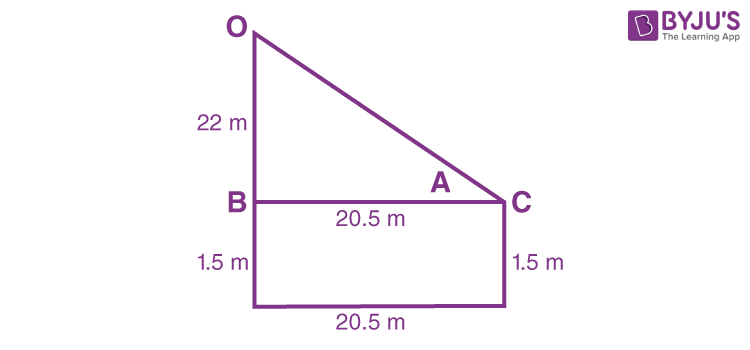# An observer 1.5 metres tall is 20.5 metres away from a tower 22 metres high. Determine the angle of elevation of the top of the tower from the eye of the observer.As per the given condition and figure drawn,

tan A = OB/BC      [Tan A = Opposite side to angle A/Adjacent side]

OB = 22 – 1.5 = 20.5 meters

BC = 20.5

Therefore,

tan A = 20.5/20.5 = 1 …….(i)

As we know, by the trigonometry values,

tan 45° = 1    ……..(ii)

Therefore, from eq.(i) and (ii), we get;

A = 45°

Hence, the angle of elevation is 45°.